Courses

Gauss' Law - Electrostatics, Electromagnetic Theory, CSIR-NET Physical Sciences Notes | Study Physics for IIT JAM, UGC - NET, CSIR NET - Physics

Physics: Gauss' Law - Electrostatics, Electromagnetic Theory, CSIR-NET Physical Sciences Notes | Study Physics for IIT JAM, UGC - NET, CSIR NET - Physics

The document Gauss' Law - Electrostatics, Electromagnetic Theory, CSIR-NET Physical Sciences Notes | Study Physics for IIT JAM, UGC - NET, CSIR NET - Physics is a part of the Physics Course Physics for IIT JAM, UGC - NET, CSIR NET.
All you need of Physics at this link: Physics

The total of the electric flux out of a closed surface is equal to the charge enclosed divided by the permittivity.

The electric flux through an area is defined as the electric field multiplied by the area of the surface projected in a plane perpendicular to the field. Gauss's Law is a general law applying to any closed surface. It is an important tool since it permits the assessment of the amount of enclosed charge by mapping the field on a surface outside the charge distribution. For geometries of sufficient symmetry, it simplifies the calculation of the electric field.

Another way of visualizing this is to consider a probe of area A which can measure the electric field perpendicular to that area. If it picks any closed surface and steps over that surface, measuring the perpendicular field times its area, it will obtain a measure of the net electric charge within the surface, no matter how that internal charge is configured.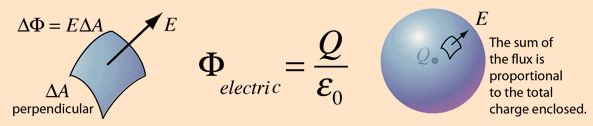Gauss' Law, Integral Form

The area integral of the electric field over any closed surface is equal to the net chargeenclosed in the surface divided by the permittivity of space. Gauss' law is a form of one of Maxwell's equations, the four fundamental equations for electricity and magnetism.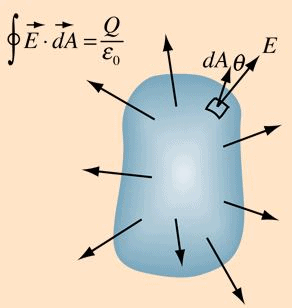Gauss' law permits the evaluation of the electric field in many practical situations by forming a symmetric Gaussian surface surrounding a charge distribution and evaluating the electric flux through that surface.

Electric Flux

The concept of electric flux is useful in association with Gauss' law. The electric flux through a planar area is defined as the electric field times the component of the area perpendicular to the field. If the area is not planar, then the evaluation of the flux generally requires an area integralsince the angle will be continually changing.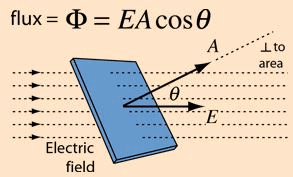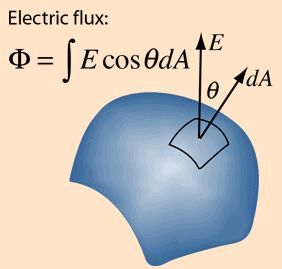When the area A is used in a vector operation like this, it is understood that the magnitude of the vector is equal to the area and the direction of the vector is perpendicular to the area.

Applications of Gauss' Law

Gauss' law is a powerful tool for the calculation of electric fields when they originate from charge distributions of sufficient symmetry to apply it.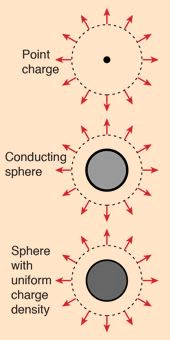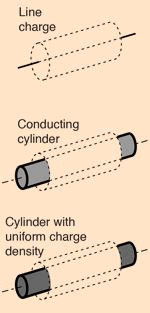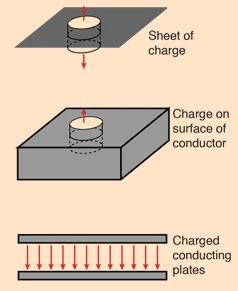If the charge distribution lacks sufficient symmetry for the application of Gauss' law, then the field must be found by summing the point charge fields of individual charge elements.

Examples are:

Electric Field of Line Charge

The electric field of a line of charge can be found by superposing the point charge fields of infinitesmal charge elements. The radial part of the field from a charge element is given by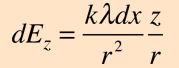The integral required to obtain the field expression is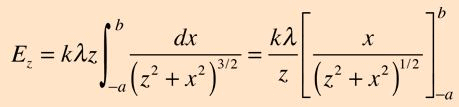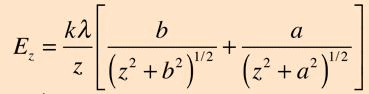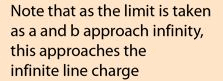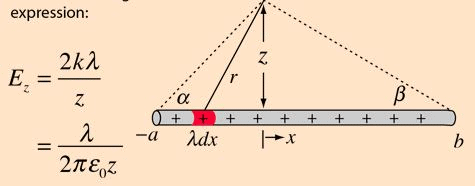Electric Field: Ring of Charge

The electric field of a ring of charge on the axis of the ring can be found by superposing the point charge fields of infinitesmal charge elements. The ring field can then be used as an element to calculate the electric field of a charged disc.

The electric fields in the xy plane cancel by symmetry, and the z-components from charge elements can be simply added.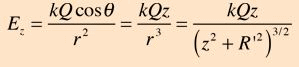Q = total Charge

If the charge is characterized by an area density and the ring by an incremental width dR' , then: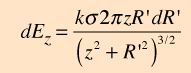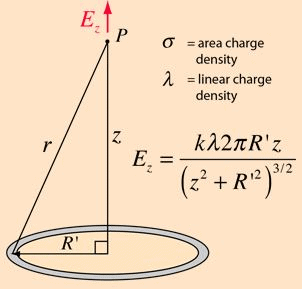This is a suitable element for the calculation of the electric field of a charged disc.

Electric Field:Disc of Charge

The electric field of a disc of charge can be found by superposing the point charge fields of infinitesmal charge elements. This can be facilitated by summing the fields of charged rings. The integral over the charged disc takes the form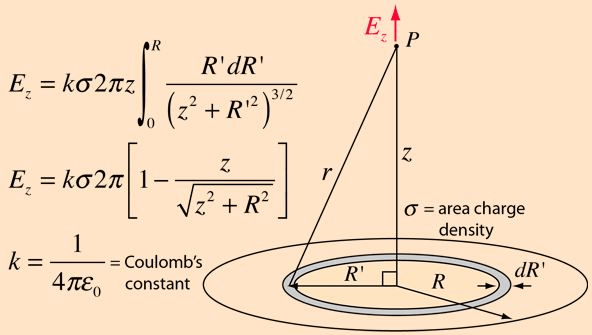The document Gauss' Law - Electrostatics, Electromagnetic Theory, CSIR-NET Physical Sciences Notes | Study Physics for IIT JAM, UGC - NET, CSIR NET - Physics is a part of the Physics Course Physics for IIT JAM, UGC - NET, CSIR NET.
All you need of Physics at this link: PhysicsUse Code STAYHOME200 and get INR 200 additional OFF Use Coupon CodePhysics for IIT JAM, UGC - NET, CSIR NET

159 docs

Track your progress, build streaks, highlight & save important lessons and more!

,

,

,

,

,

,

,

,

,

,

,

,

,

,

,

,

,

,

,

,

,

,

,

,

,

,

,

,

,

,

,

,

,

;